Get inspired by the success stories of our students in IIT JAM MS, ISI  MStat, CMI MSc DS.  Learn More

# ISI MStat PSB 2013 Problem 5 | Simple Random SamplingThis is a sample problem from ISI MStat PSB 2013 Problem 5. It is based on the simple random sampling model, finding the unbiased estimates of the population size. But think over the "Food for Thought" any kind of discussion will be appreciated. Give it a try!

## Problem- ISI MStat PSB 2013 Problem 5

A box has a unknown number of tickets serially numbered 1,2,.....,N. Two tickets are drawn using simple random sampling without replacement (SRSWOR) from the box. If X and Y are the numbers on the tickets and Z=max(X,Y), show that

(a) Z is not ubiased for N.

(b)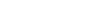is unbiased for N if and only if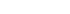and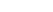.

### Prerequisites

Naive Probability

Counting priciples

Unbiased estimators.

Simple random sampling .

## Solution :

For this problem, first let us find the pmf of Z, where we will need some counting techniques.

Since we are drawing balls at a random and not replacing the drawn ball after each draw (SRSWOR), so, clearly its about choosing two numbers from the se of N elements {1,.....,N}. So, all possible sample of size 2 , that than be drawn from the population of N units is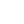.

now Z defined here as the maximum of the two chosen numbers, so, all possible values of Z are 2,3,....,N.

Now lets assume that Z=k, so now we just need to find out what are the possible pairs, such that k comes the max among both, or in other words if k is the maximum of the drawn numbers, what are the possible values that the other number can take ? Well, its simple the other ticket can carry any number less than k, an since there are k-1 such numbers. So there are (k-1) such pairs where the maximum numbered ticket is k. (not concerned on the ordering, of the two observation)

So, the pmf of Z=max(X,Y) , i.e.(a) So, now to check whether Z is unbiased for N, we need to check E(Z),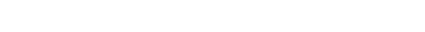.

so,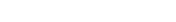. Hence Z is not Unbiased for the population size, N.

(b) Similarly, we find the expectation of T=aX+bY+c,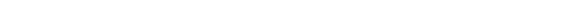now here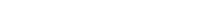, so,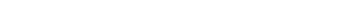clearly, E(T) = N, i.e T will be unbiased for N, iff a+b=2 and c=-1.

Hence we are done !

## Food For Thought

Now, suppose that the numbers on the tickets are random, that is it can be any positive integer, ( like say 220 or 284), but thankfully you now the total number of tickets .i.e. N is known . Now you are collecting tickets for yourself and k-1 of your friends, and the number c is lucky for you and you wish to keep it in your collection, and select the remaining k-1 tickets out of N-1 tickets, and you calculate a sample mean(of the collected numbers)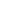, Can I claim that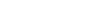is an unbiased estimator of the population total ? Do you know this estimator shows less variance than the conventional unbiased estimator of the population total? Can you show that too?? why do you think the variance minimizes??

By the Way, Do you know, in mathematics 220 and 284 are quite special ?? They are the first "amicable numbers". One can obtain the other by summing over its own divisors !! So, to become amicable one needs to increase the size of their mind and heart !! Keep increasing both!! Till then.... bye.

## Subscribe to Cheenta at Youtube

This is a sample problem from ISI MStat PSB 2013 Problem 5. It is based on the simple random sampling model, finding the unbiased estimates of the population size. But think over the "Food for Thought" any kind of discussion will be appreciated. Give it a try!

## Problem- ISI MStat PSB 2013 Problem 5

A box has a unknown number of tickets serially numbered 1,2,.....,N. Two tickets are drawn using simple random sampling without replacement (SRSWOR) from the box. If X and Y are the numbers on the tickets and Z=max(X,Y), show that

(a) Z is not ubiased for N.

(b)is unbiased for N if and only ifand.

### Prerequisites

Naive Probability

Counting priciples

Unbiased estimators.

Simple random sampling .

## Solution :

For this problem, first let us find the pmf of Z, where we will need some counting techniques.

Since we are drawing balls at a random and not replacing the drawn ball after each draw (SRSWOR), so, clearly its about choosing two numbers from the se of N elements {1,.....,N}. So, all possible sample of size 2 , that than be drawn from the population of N units is.

now Z defined here as the maximum of the two chosen numbers, so, all possible values of Z are 2,3,....,N.

Now lets assume that Z=k, so now we just need to find out what are the possible pairs, such that k comes the max among both, or in other words if k is the maximum of the drawn numbers, what are the possible values that the other number can take ? Well, its simple the other ticket can carry any number less than k, an since there are k-1 such numbers. So there are (k-1) such pairs where the maximum numbered ticket is k. (not concerned on the ordering, of the two observation)

So, the pmf of Z=max(X,Y) , i.e.(a) So, now to check whether Z is unbiased for N, we need to check E(Z),.

so,. Hence Z is not Unbiased for the population size, N.

(b) Similarly, we find the expectation of T=aX+bY+c,now here, so,clearly, E(T) = N, i.e T will be unbiased for N, iff a+b=2 and c=-1.

Hence we are done !

## Food For Thought

Now, suppose that the numbers on the tickets are random, that is it can be any positive integer, ( like say 220 or 284), but thankfully you now the total number of tickets .i.e. N is known . Now you are collecting tickets for yourself and k-1 of your friends, and the number c is lucky for you and you wish to keep it in your collection, and select the remaining k-1 tickets out of N-1 tickets, and you calculate a sample mean(of the collected numbers), Can I claim thatis an unbiased estimator of the population total ? Do you know this estimator shows less variance than the conventional unbiased estimator of the population total? Can you show that too?? why do you think the variance minimizes??

By the Way, Do you know, in mathematics 220 and 284 are quite special ?? They are the first "amicable numbers". One can obtain the other by summing over its own divisors !! So, to become amicable one needs to increase the size of their mind and heart !! Keep increasing both!! Till then.... bye.

## Subscribe to Cheenta at Youtube

This site uses Akismet to reduce spam. Learn how your comment data is processed.

### Knowledge Partner